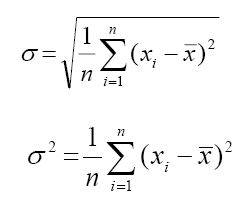# How To Find Variance From Standard Deviation

Statistics refers to the collection, analysis, interpretation and presentation of masses of numerical data. Variance and the standard deviation are important topics in statistics. Variance is a measure of central dispersion. It is the average of the squared difference from the mean. Standard deviation calculates the dispersion of a dataset relative to its mean. A standard deviation is a useful tool in investing and trading strategies. If the data points are away from the mean, there is a higher deviation within the data set. Hence, the more spread out the data, the greater the standard deviation. Mean is the average of a given set of observations. In this article, we will discuss how to find the variance from standard deviation.

## What is Variance and Standard Deviation?

The average of the squared differences from the mean is known as the variance.

Variance is denoted by σ2. The square root of the σ2 gives the standard deviation. Standard deviation calculates the dispersion of a dataset relative to its mean.

## How to Calculate Variance from Standard Deviation?

You can calculate the variance from standard deviation in a single step.
If standard deviation(σ) is given, then find the square of σ.

σ2 gives the variance.

## Formula### Solved Example

Example: The standard deviation of a set of numbers is 6.2. The variance is given by

(1) 38.04

(2) 2.48

(3) 38. 44

(4) 2.08

Solution:

Given standard deviation, σ = 6.2

So variance = σ2 = 6.22 = 38.44

Hence option (3) is the answer.